# Evaluating Reflective Measurement Model. Step 2 & 3: Reliability and Convergent Validity.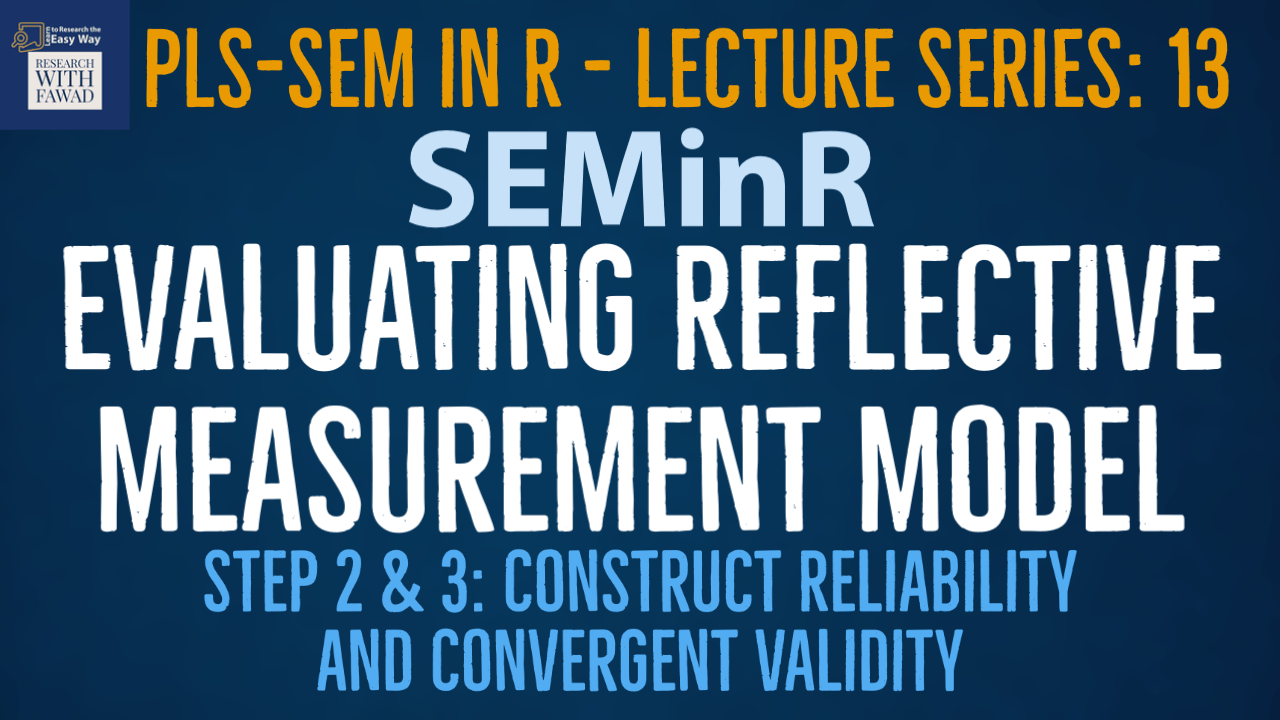### SEMinR Lecture Series

This series of lectures on SEMinR Package will focus on evaluating reflective measurement model.

## Evaluating Reflective Measurement Model

• In the last session the focus was on assessing indicator reliability.
• This lecture will focus on Step 2: Internal Consistency and Step 3: Convergent Validity
• The figure illustrates the reflective measurement model evaluation process.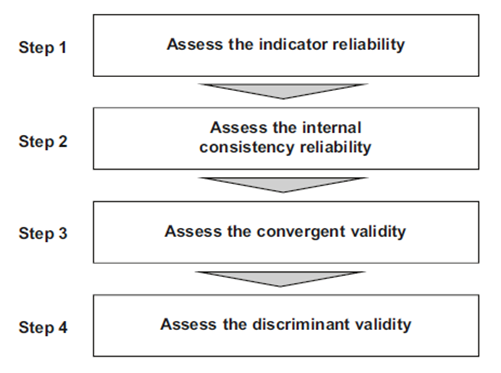## Internal Consistency Reliability

• The second step in reflective measurement model assessment involves examining internal consistency reliability.
• One of the primary measures used in PLS-SEM is Jöreskog’s (1971) composite reliability rhoc. Higher values indicate higher levels of reliability. Values over .70 are normally considered reliable.
• Reliability values between 0.60 and 0.70 are considered “acceptable in exploratory research,” whereas values between 0.70 and 0.90 range from “satisfactory to good.”
• Values above 0.90 (and definitely above 0.95) are problematic, since they indicate that the indicators are redundant, thereby reducing construct validity (Diamantopoulos, Sarstedt, Fuchs, Wilczynski, & Kaiser, 2012).
• Reliability values of 0.95 and above also suggest the possibility of undesirable response patterns (e.g., straight-lining), thereby triggering inflated correlations among the error terms of the indicators.
• summary_simple in our example.
• Cronbach’s alpha is another measure of internal consistency reliability, which assumes the same thresholds as the composite reliability (rhoc).
• A major limitation of Cronbach’s alpha, however, is that it assumes all indicator loadings are the same in the population (also referred to as tau-equivalence). The violation of this assumption manifests itself in lower reliability values than those produced by rhoc.
• While Cronbach’s alpha is rather conservative, the composite reliability rhoc may be too liberal, and the construct’s true reliability is typically viewed as within these two extreme values.
• As an alternative and building on Dijkstra (2010), subsequent research has proposed the exact (or consistent) reliability coefficient rhoA (Dijkstra, 2014; Dijkstra & Henseler, 2015).
• The reliability coefficient rhoA usually lies between the conservative Cronbach’s alpha and the liberal composite reliability and is therefore considered and acceptable compromise between these two measures.
• To evaluate the composite reliability of the construct measures, inspect the summary_simple object by using \$reliability:

``````#Inspect the composite reliability
summary_simple\$reliability``````
• The internal consistency reliability values are displayed in a matrix format. With rhoA values in between Alpha and Composite Reliability.
• Similarly, the results for Cronbach’s alpha and Composite Reliability are above the 0.70 threshold (Hair et al., 2019), indicating that all construct measures are reliable.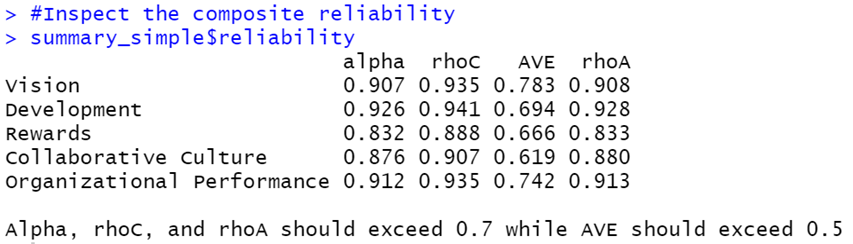## Reliability Plots

• The results can also be visualized using a bar chart, requested by the plot() function on the summary_simple\$reliability object.
• This plot visualizes the reliability in terms of Cronbach’s alpha, rhoA, and rhoC for all constructs. Note that the plots will be outputted to the plots panel window in Rstudio.
``````#Plot the reliabilities of constructs
plot(summary_simple\$reliability)``````
• The horizontal dashed blue line indicates the common minimum threshold level for the three reliability measures (i.e., 0.70).
• As indicated in Fig. all Cronbach’s alpha, rhoA, and rhoC values exceed the threshold.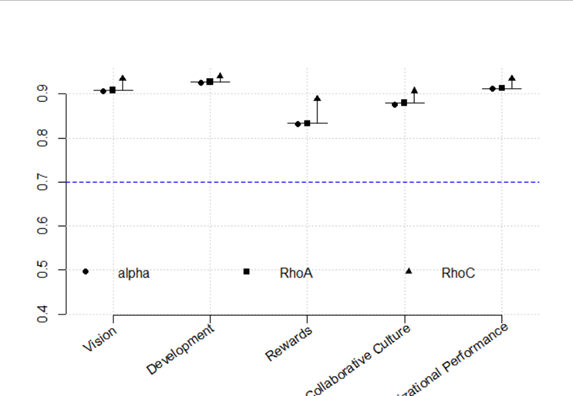## Convergent Validity

• The third step is to assess (the) convergent validity of each construct. Convergent validity is the extent to which the construct converges in order to explain the variance of its indicators.
• The metric used for evaluating a construct’s convergent validity is the average variance extracted (AVE) for all indicators on each construct.
• The AVE is defined as the grand mean value of the squared loadings of the indicators associated with the construct (i.e., the sum of the squared loadings divided by the number of indicators).
• Therefore, the AVE is equivalent to the communality of a construct. The minimum acceptable AVE is 0.50 – an AVE of 0.50 or higher indicates the construct explains 50 percent or more of the indicators’ variance that make up the construct (Hair et al., 2022).
• Convergent validity assessment is based on the average variance extracted (AVE) values (Hair et al., 2019), which can also be accessed by summary_simple\$reliability. . Figure shows the AVE values along with the internal consistency reliability values.
• In this example, the AVE values are well above the required minimum level of 0.50 (Hair et al., 2019). Thus, the measures of the three reflectively measured constructs have high levels of convergent validity.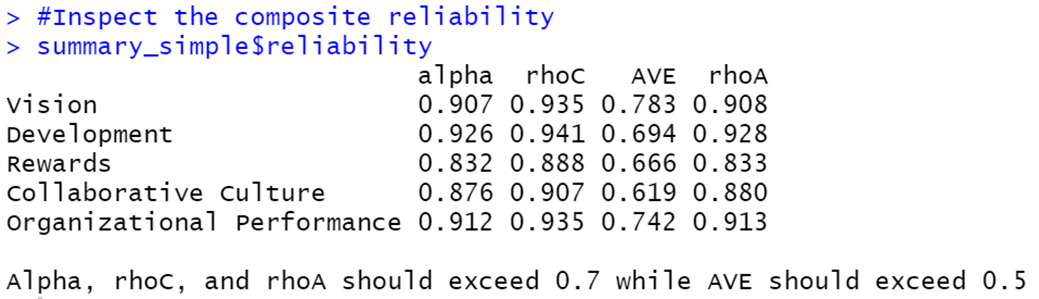## Complete Code

``````library(seminr)
# Create measurement model
#For Multi_Items Identified Separately
#composite("Vision", c("VIS1","VIS2","VIS3","VIS4")
simple_mm <- constructs(
composite("Vision", multi_items("VIS", 1:4)),
composite("Development", multi_items("DEV", 1:7)),
composite("Rewards", multi_items("RW",1:4)),
composite("Collaborative Culture", multi_items("CC", 1:6)),
composite("Organizational Performance", multi_items("OP", 1:5)))
# Create structural model
simple_sm <- relationships(
paths(from = c("Vision","Development","Rewards"), to = "Collaborative Culture"),
paths(from = "Collaborative Culture", to = "Organizational Performance"))
# Estimate the model
simple_model <- estimate_pls(data = datas,
measurement_model = simple_mm,
structural_model = simple_sm)
# Summarize the model results
summary_simple <- summary(simple_model)
#To Display all the contents in the summary_simple Object
summary_simple
# Iterations to converge
summary_simple\$iterations

# Inspect the indicator reliability

# Write the indicator reliability to csv file

#Inspect the composite reliability
summary_simple\$reliability
write.csv(x = summary_simple\$reliability, file = "reliability.csv")
``````

## Reference

Hair Jr, J. F., Hult, G. T. M., Ringle, C. M., Sarstedt, M., Danks, N. P., & Ray, S. (2021). Partial Least Squares Structural Equation Modeling (PLS-SEM) Using R: A Workbook.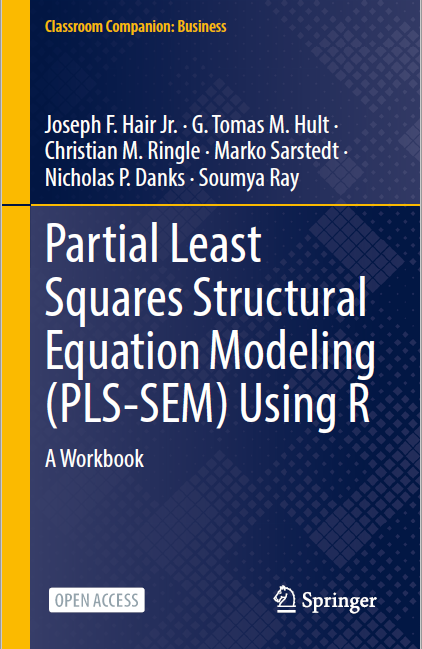The tutorials on SEMinR are based on the mentioned book. The book is open source and available for download under this link.